## How long does it take electrons to get from a car battery to the starting motor? Assume the current is 300 A and the electrons travel throug

Question

How long does it take electrons to get from a car battery to the starting motor? Assume the current is 300 A and the electrons travel through a copper wire with cross-sectional area 0.250 cm2 and length 0.900 m. The number of charge carriers per unit volume is 8.49 × 1028 m-3.

in progress 0
6 months 2021-08-18T12:13:40+00:00 2 Answers 1 views 0

16.98 minutes

Explanation:

We are given that

Current,I=300 A

Area, A=0.250 square cm=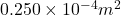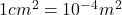Length of wire,d=0.9 m

Number of charge carriers per unit volume=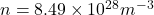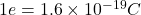We know that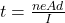Using the formula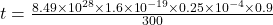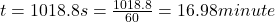Explanation:

current, i = 300 A

Area of crossection, A = 0.250 cm² = 0.250 x 10^-4 m²

length, l = 0.9 m

number of electrons per unit volume, n = 8.49 x 10^28 /m³

let v be the drift velocity and t be the time taken by the electrons

i = n e A v

300 = 8.49 x 10^28 x 1.6 x 10^-19 x 0.25 x 10^-4 x v

v = 8.84 x 10^-4 m/s

Time, t = distance / velocity

t = 0.9 / (8.84 x 10^-4)

t = 1018.8 s

t = 17 minutes